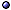Symplectic Structures

Symplectic Vector Space
$Def: A vector space V with a non-degenerate antisymmetric rank-2 tensor Ω. * Symmetry group: The Lie group preserving this structure is the symplectic group Sp(V, Ω) ⊂ GL(V). * Subspaces: W < V is Isotropic if WW:= {null vectors of Ω|W}; Coisotropic (first class) if WW; Weakly symplectic (second class) if WW = 0; Lagrangian if isotropic and coisotropic, W = W; Otherwise, it is called mixed. Canonical Transformation > s.a. fock space; quantum states.$ Def: Given a symplectic vector space (V, Ω), a canonical transformation is a linear map A: VV that preserves Ω, i.e., Ω(A(X), A(Y)) = Ω(X, Y), for all X, YV, or At Ω A = Ω.
* Generating function: The function

H(X) = $$-{1\over2}$$X At Ω X .

* Point transformation: A canonical transformation that does not mix coordinates and momenta.
@ Generating functions: Anselmi EPJC(16)-a1511 [in classical mechanics and quantum field theory]; Anselmi EPJC(16)-a1604 [reference formulas].
@ Special types: Brodlie JMP(04)qp [non-linear]; Dereli et al IJMPA(09)-a0904 [in 3D phase space with Nambu bracket].
@ Quantum: Bordner JMP(97); Davis & Ghandour PLA(01) [and system equivalence]; Cervero & Rodríguez IJTP(02)qp/01 [and squeezing]; Lacki SHPMP(04) [in early quantum mechanics].
@ Generalizations: Cariñena et al JGM(13)-a1303 [canonoid transformations, and symmetries]; Kupsch RVMP(14) [for fermions]; Valtancoli JMP(15)-a1510 [with non-commutative Poisson brackets, minimal length].

Kähler Spaces and Manifolds / Structures > s.a. complex structure.
$Kähler space: A symplectic vector space (V, Ω) with a complex structure J, compatible with Ω in the sense that the rank-2 tensor ΩJ is positive-definite and symmetric (a Kähler metric).$ Kähler manifold: A triple (M, Ω, J), with M a differentiable manifold, Ω and J strongly compatible, and J integrable; > s.a. Wikipedia page.
@ Kähler space: in Artin 57; Flaherty 76 [in relativity]; in Helgason 78; Calabi & Chen JDG(02) [space of Kähler metrics].
@ Kähler manifold: Hashimoto et al JMP(97) [hyperkähler metrics from Ashtekar variables]; Pedersen et al LMP(99) [quasi-Einstein Kähler metrics]; Chen JDG(00) [space of Kähler metrics]; Cortés m.DG/01-ln [special Kähler manifolds]; Ross & Thomas JDG(06) [constant curvature, obstructions]; Aazami & Maschler a1711 [from Lorentzian geometries].
@ Generalizations: Gualtieri CMP(14) [generalized complex geometry]; > s.a. Hyperkähler Structure.

Generalizations > s.a. Contact Manifold; Cosymplectic Structures; types of symplectic structures.
* Higher-order structures: A manifold is n-plectic if it is equipped with a closed, non-degenerate form of degree n + 1; > s.a. formulations of general relativity.
@ General references: Zumino MPLA(91) [Fermionic coordinates]; Nikolić qp/98 [non equal-time]; Vanhecke BJP(06)mp/05 [non-commutative configuration space]; Sergyeyev Sigma(07)mp/06 [weakly non-local]; > s.a. non-commutative physics; poisson structures [Moyal and Nambu brackets]; Supermanifolds.
@ Higher-order structures: Rogers PhD(11)-a1106; Sämann & Szabo RVMP(13)-a1211 [quantization of 2-plectic manifolds].

Related Conceptssee symplectic manifolds; symplectic structures in physics.